# 八、图像分割

*本章涉及的主题如下：

• 图像中的 Hough 变换圆和线检测（带scikit-image
• 阈值化和大津分割（带scikit-image
• 基于边缘/基于区域的分割技术（带scikit-image
• Felzenszwalb、SLIC、QuickShift 和紧凑分水岭算法（带scikit-image
• 活动轮廓。。。

# 什么是图像分割？

import numpy as np
from skimage.transform import (hough_line, hough_line_peaks, hough_circle, hough_circle_peaks)
from skimage.draw import circle_perimeter
from skimage.feature import canny
from skimage.data import astronaut
from skimage.color import rgb2gray, gray2rgb, label2rgb
from skimage import img_as_float
from skimage.morphology import skeletonize
from skimage import data, img_as_float
import matplotlib.pyplot as pylab
from matplotlib import cm
from skimage.filters import sobel, threshold_otsu
from skimage.feature import canny
from skimage.segmentation import felzenszwalb, slic, quickshift, watershed
from skimage.segmentation import mark_boundaries, find_boundaries


# 阈值化与大津分割

image = rgb2gray(imread('../images/horse.jpg'))
thresh = threshold_otsu(image)
binary = image > thresh

fig, axes = pylab.subplots(nrows=2, ncols=2, figsize=(20, 15))
axes = axes.ravel()
axes, axes = pylab.subplot(2, 2, 1), pylab.subplot(2, 2, 2)
axes = pylab.subplot(2, 2, 3, sharex=axes, sharey=axes)
axes = pylab.subplot(2, 2, 4, sharex=axes, sharey=axes)
axes.imshow(image, cmap=pylab.cm.gray)
axes.set_title('Original', size=20), axes.axis('off')
axes.hist(image.ravel(), bins=256, normed=True)
axes.set_title('Histogram', size=20), axes.axvline(thresh, color='r')
axes.imshow(binary, cmap=pylab.cm.gray)
axes.set_title('Thresholded (Otsu)', size=20), axes.axis('off')
axes.axis('off'), pylab.tight_layout(), pylab.show()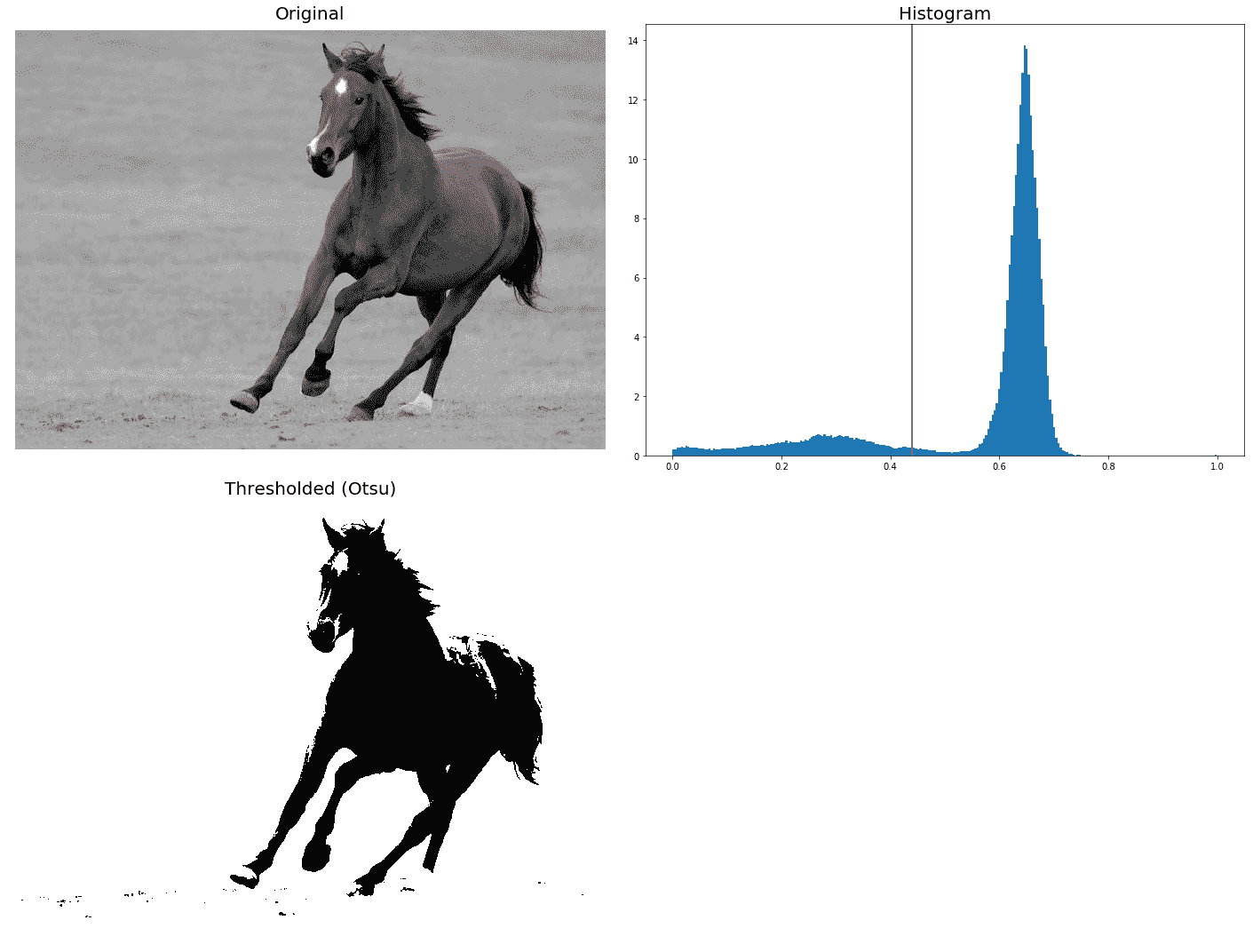# 基于边缘/基于区域的分割

coins = data.coins()hist = np.histogram(coins, bins=np.arange(0, 256), normed=True)fig, axes = pylab.subplots(1, 2, figsize=(20, 10))axes.imshow(coins, cmap=pylab.cm.gray, interpolation='nearest')axes.axis('off'), axes.plot(hist[:-1], hist, lw=2)axes.set_title('histogram of gray values') ...


# 基于边缘的分割

edges = canny(coins, sigma=2)
fig, axes = pylab.subplots(figsize=(10, 6))
axes.imshow(edges, cmap=pylab.cm.gray, interpolation='nearest')
axes.set_title('Canny detector'), axes.axis('off'), pylab.show()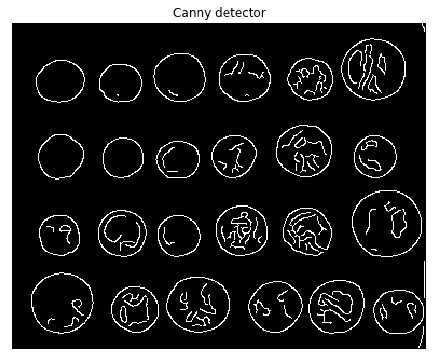from scipy import ndimage as ndi
fill_coins = ndi.binary_fill_holes(edges)
fig, axes = pylab.subplots(figsize=(10, 6))
axes.imshow(fill_coins, cmap=pylab.cm.gray, interpolation='nearest')
axes.set_title('filling the holes'), axes.axis('off'), pylab.show()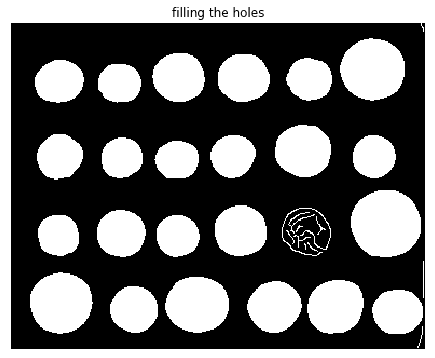from skimage import morphology
coins_cleaned = morphology.remove_small_objects(fill_coins, 21)
fig, axes = pylab.subplots(figsize=(10, 6))
axes.imshow(coins_cleaned, cmap=pylab.cm.gray, interpolation='nearest')
axes.set_title('removing small objects'), axes.axis('off'), pylab.show()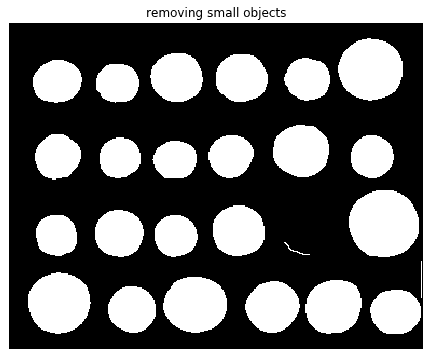# 形态分水岭算法

• 找到标记和分割标准（用于分割区域的函数，通常是对比度/梯度）
• 使用这两个元素运行标记控制的分水岭算法

elevation_map = sobel(coins)
fig, axes = pylab.subplots(figsize=(10, 6))
axes.imshow(elevation_map, cmap=pylab.cm.gray, interpolation='nearest')
axes.set_title('elevation map'), axes.axis('off'), pylab.show()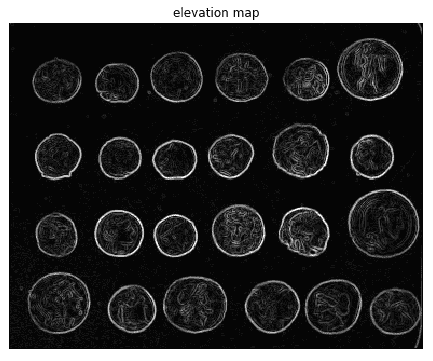markers = np.zeros_like(coins)
markers[coins < 30] = 1
markers[coins > 150] = 2
print(np.max(markers), np.min(markers))
fig, axes = pylab.subplots(figsize=(10, 6))
a = axes.imshow(markers, cmap=plt.cm.hot, interpolation='nearest')
plt.colorbar(a)
axes.set_title('markers'), axes.axis('off'), pylab.show()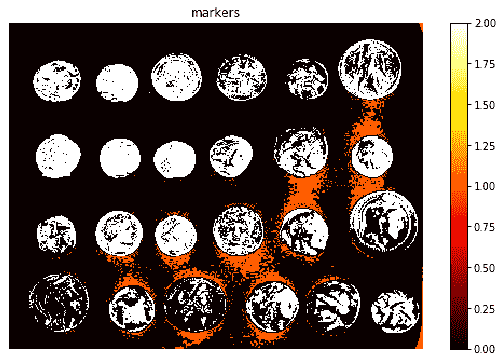segmentation = morphology.watershed(elevation_map, markers)
fig, axes = pylab.subplots(figsize=(10, 6))
axes.imshow(segmentation, cmap=pylab.cm.gray, interpolation='nearest')
axes.set_title('segmentation'), axes.axis('off'), pylab.show()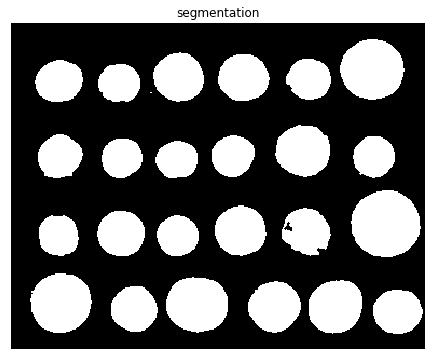segmentation = ndi.binary_fill_holes(segmentation - 1)
labeled_coins, _ = ndi.label(segmentation)
image_label_overlay = label2rgb(labeled_coins, image=coins)
fig, axes = pylab.subplots(1, 2, figsize=(20, 6), sharey=True)
axes.imshow(coins, cmap=pylab.cm.gray, interpolation='nearest')
axes.contour(segmentation, [0.5], linewidths=1.2, colors='y')
axes.imshow(image_label_overlay, interpolation='nearest')
for a in axes:
a.axis('off')
pylab.tight_layout(), pylab.show()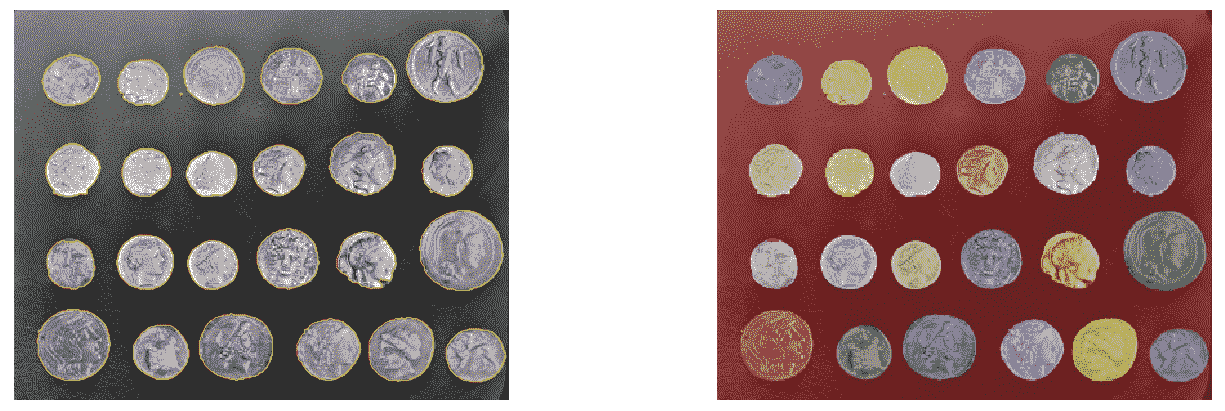# Felzenszwalb 基于图的高效图像分割

Felzenszwalb 的算法采用基于图形的分割方法。它首先构造一个无向图，其中图像像素作为顶点（要分割的集合），两个顶点之间的边的权重是相异性的某种度量（例如，强度的差异）。在基于图的方法中，将图像划分为多个部分的问题转化为在构造的图中查找连接的组件。同一组件中两个顶点之间的边应具有相对较低的权重，而不同组件中顶点之间的边应具有较高的权重。

from matplotlib.colors import LinearSegmentedColormap
for imfile in ['../images/eagle.png', '../images/horses.png', '../images/flowers.png', '../images/bisons.png']:
pylab.figure(figsize=(20,10))
segments_fz = felzenszwalb(img, scale=100, sigma=0.5, min_size=400)
borders = find_boundaries(segments_fz)
unique_colors = np.unique(segments_fz.ravel())
segments_fz[borders] = -1
colors = [np.zeros(3)]
for color in unique_colors:
colors.append(np.mean(img[segments_fz == color], axis=0))
cm = LinearSegmentedColormap.from_list('pallete', colors, N=len(colors))
pylab.subplot(121), pylab.imshow(img), pylab.title('Original', size=20), pylab.axis('off')
pylab.subplot(122), pylab.imshow(segments_fz, cmap=cm),
pylab.title('Segmented with Felzenszwalbs\'s method', size=20), pylab.axis('off')
pylab.show()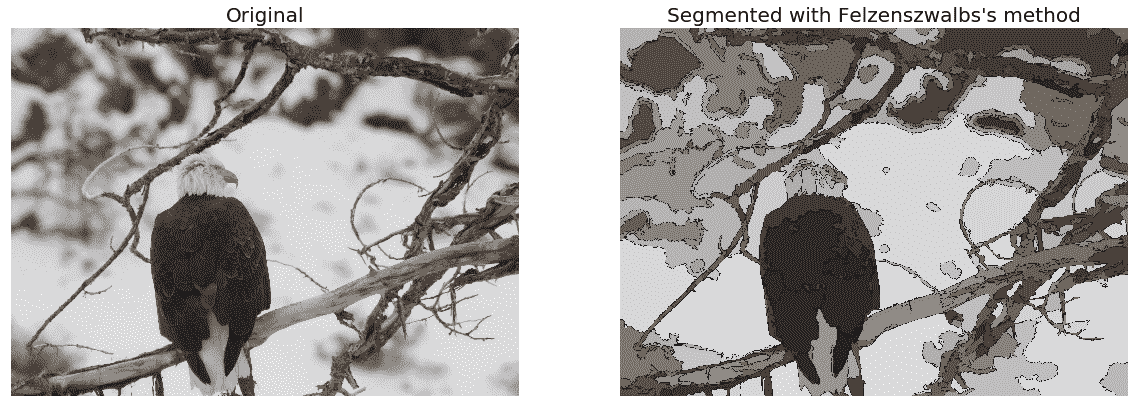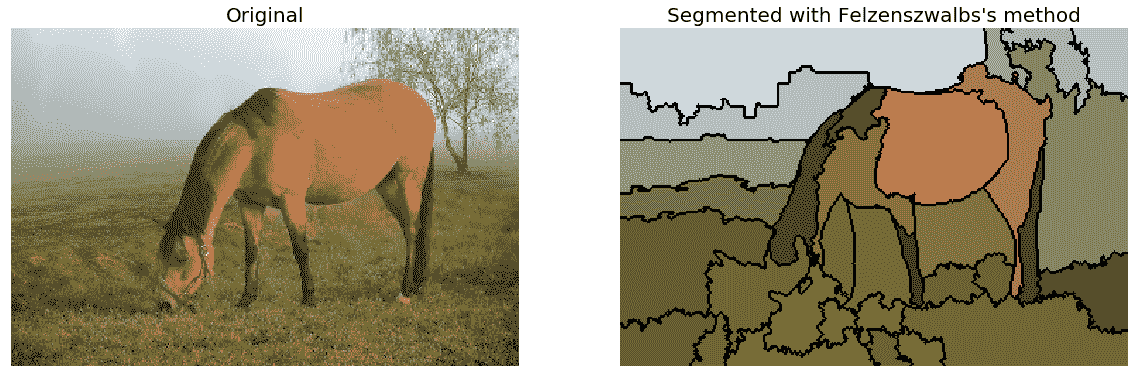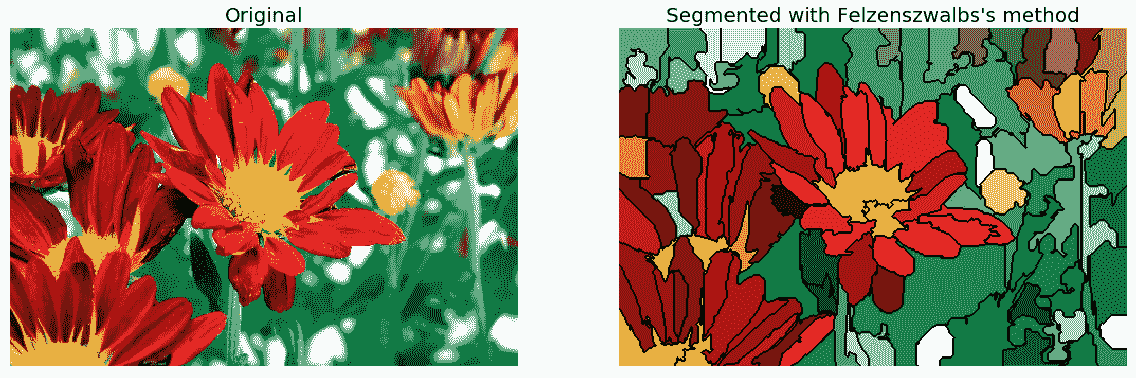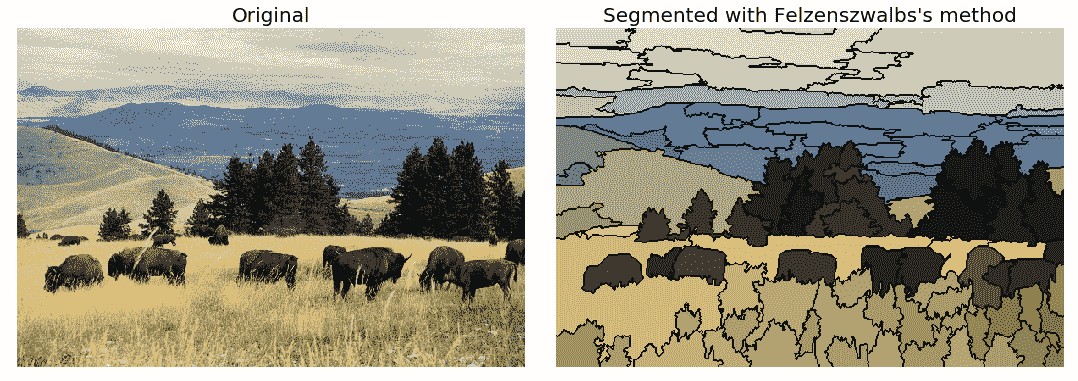def plot_image(img, title):
pylab.imshow(img), pylab.title(title, size=20),  pylab.axis('off')

pylab.figure(figsize=(15,10))
i = 1
for scale in [50, 100, 200, 400]:
plt.subplot(2,2,i)
segments_fz = felzenszwalb(img, scale=scale, sigma=0.5, min_size=200)
plot_image(mark_boundaries(img, segments_fz, color=(1,0,0)), 'scale=' + str(scale))
i += 1
pylab.suptitle('Felzenszwalbs\'s method', size=30), pylab.tight_layout(rect=[0, 0.03, 1, 0.95])
pylab.show()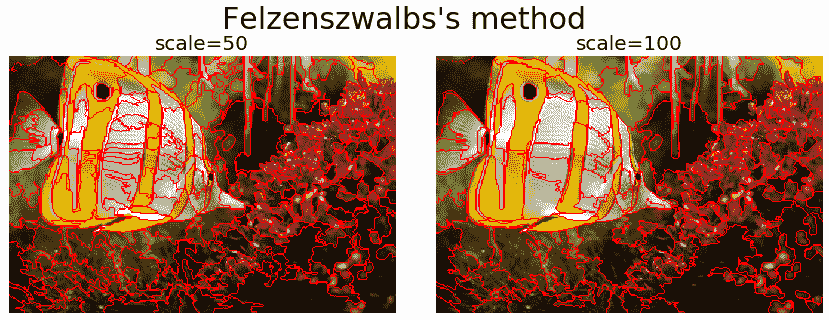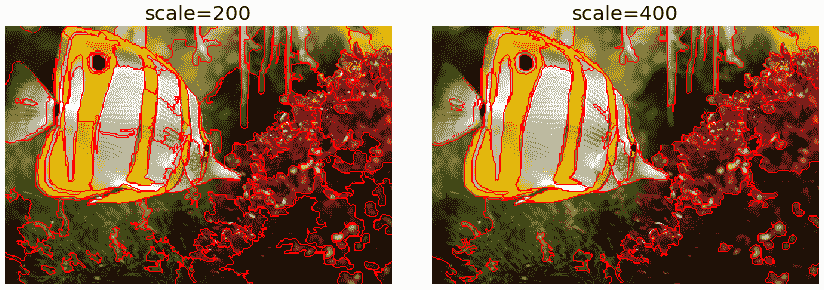# SLIC

SLIC 算法只是在颜色空间（RGB 或 Lab）和图像位置（即像素坐标：x的五维空间中进行 k 均值聚类（我们将在第 9 章图像处理中的经典机器学习方法中对该聚类算法进行更多探讨），y。该算法非常有效，因为聚类方法更简单。要使用该算法获得良好的结果，必须在实验室颜色空间中工作。该算法已迅速获得势头，目前已被广泛使用。紧致度参数权衡颜色相似性和接近性，而n_segments参数选择 k-means 的中心数。下一个代码块演示如何使用scikit-image ...实现该算法

# 碎布合并

from skimage import segmentation
from skimage.future import graph

def _weight_mean_color(graph, src, dst, n):
diff = graph.node[dst]['mean color'] - graph.node[n]['mean color']
diff = np.linalg.norm(diff)
return {'weight': diff}

def merge_mean_color(graph, src, dst):
graph.node[dst]['total color'] += graph.node[src]['total color']
graph.node[dst]['pixel count'] += graph.node[src]['pixel count']
graph.node[dst]['mean color'] = (graph.node[dst]['total color'] /  graph.node[dst]['pixel count'])

labels = segmentation.slic(img, compactness=30, n_segments=400)
g = graph.rag_mean_color(img, labels)
labels2 = graph.merge_hierarchical(labels, g, thresh=35, rag_copy=False,
in_place_merge=True,
merge_func=merge_mean_color,
weight_func=_weight_mean_color)
out = label2rgb(labels2, img, kind='avg')
out = segmentation.mark_boundaries(out, labels2, (0, 0, 0))
pylab.figure(figsize=(20,10))
pylab.subplot(121), pylab.imshow(img), pylab.axis('off')
pylab.subplot(122), pylab.imshow(out), pylab.axis('off')
pylab.tight_layout(), pylab.show()# 快速换档

QuickShift是一种二维图像分割算法，基于核化均值漂移算法的近似值，相对较新。它属于局部（非参数）模式搜索算法家族（基于将每个数据点关联到潜在概率密度函数模式的思想），并应用于由颜色空间和图像位置组成的 5D 空间。

QuickShift 实际上同时计算多个尺度的分层分割，这是该算法的优点之一。QuickShift 有两个主要参数：参数 sigma 控制局部密度近似的比例，其中参数max_dist选择分层分割中的一个级别。。。

# 紧凑流域

from skimage.segmentation import watershed
pylab.figure(figsize=(15,10))
i = 1
for markers in [200, 1000]:
for compactness in [0.001, 0.0001]:
pylab.subplot(2,2,i)
plot_image(mark_boundaries(img, segments_watershed, color=(1,0,0), 'markers=' + str(markers) + '.compactness=' + str(compactness))
i += 1
pylab.suptitle('Compact watershed', size=30), pylab.tight_layout(rect=[0, 0.03, 1, 0.95]), pylab.show()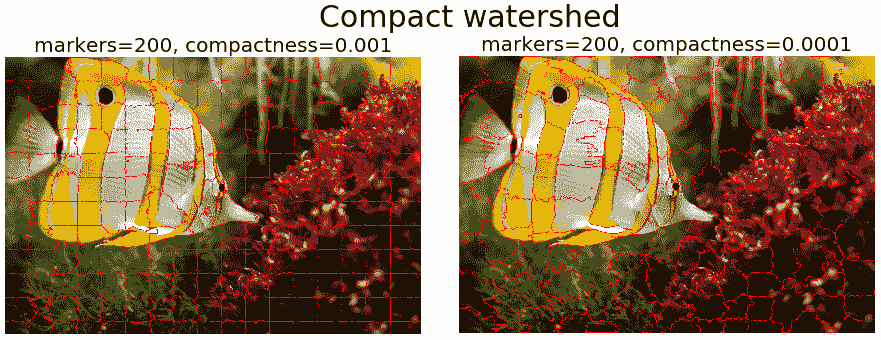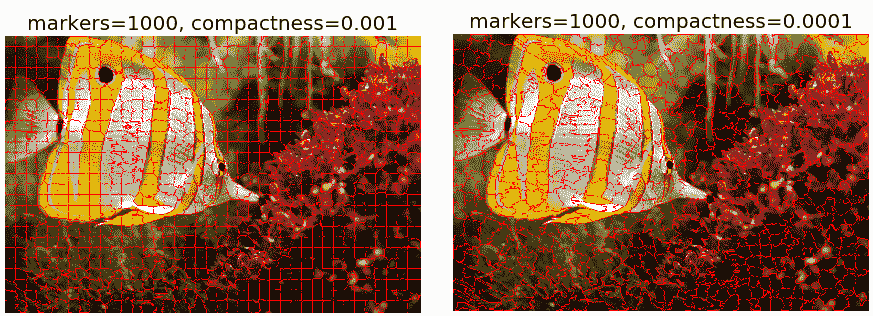# 形态蛇

from skimage.segmentation import (morphological_chan_vese, morphological_geodesic_active_contour,

def store_evolution_in(lst):
"""Returns a callback function to store the evolution of the level sets in
the given list.
"""

def _store(x):
lst.append(np.copy(x))
return _store

# Morphological ACWE
image_gray = rgb2gray(image)
# initial level set
init_lvl_set = checkerboard_level_set(image_gray.shape, 6)
# list with intermediate results for plotting the evolution
evolution = []
callback = store_evolution_in(evolution)
lvl_set = morphological_chan_vese(image_gray, 30, init_level_set=init_lvl_set, smoothing=3, iter_callback=callback)
fig, axes = pylab.subplots(2, 2, figsize=(8, 6))
axes = axes.flatten()
axes.imshow(image, cmap="gray"), axes.set_axis_off(), axes.contour(lvl_set, [0.5], colors='r')
axes.set_title("Morphological ACWE segmentation", fontsize=12)
axes.imshow(lvl_set, cmap="gray"), axes.set_axis_off()
contour = axes.contour(evolution, [0.5], colors='g')
contour.collections.set_label("Iteration 5")
contour = axes.contour(evolution, [0.5], colors='y')
contour.collections.set_label("Iteration 10")
contour = axes.contour(evolution[-1], [0.5], colors='r')
contour.collections.set_label("Iteration " + str(len(evolution)-1))
axes.legend(loc="upper right"), axes.set_title("Morphological ACWE evolution", fontsize=12)

# Morphological GAC
image_gray = rgb2gray(image)
# initial level set
init_lvl_set = np.zeros(image_gray.shape, dtype=np.int8)
init_lvl_set[10:-10, 10:-10] = 1
# list with intermediate results for plotting the evolution
evolution = []
callback = store_evolution_in(evolution)
lvl_set = morphological_geodesic_active_contour(gimage, 400, init_lvl_set, smoothing=1, balloon=-1,
threshold=0.7, iter_callback=callback)
axes.imshow(image, cmap="gray"), axes.set_axis_off(), axes.contour(lvl_set, [0.5], colors='r')
axes.set_title("Morphological GAC segmentation", fontsize=12)
axes.imshow(lvl_set, cmap="gray"), axes.set_axis_off()
contour = axes.contour(evolution, [0.5], colors='g')
contour.collections.set_label("Iteration 100")
contour = axes.contour(evolution, [0.5], colors='y')
contour.collections.set_label("Iteration 200")
contour = axes.contour(evolution[-1], [0.5], colors='r')
contour.collections.set_label("Iteration " + str(len(evolution)-1))
axes.legend(loc="upper right"), axes.set_title("Morphological GAC evolution", fontsize=12)
fig.tight_layout(), pylab.show()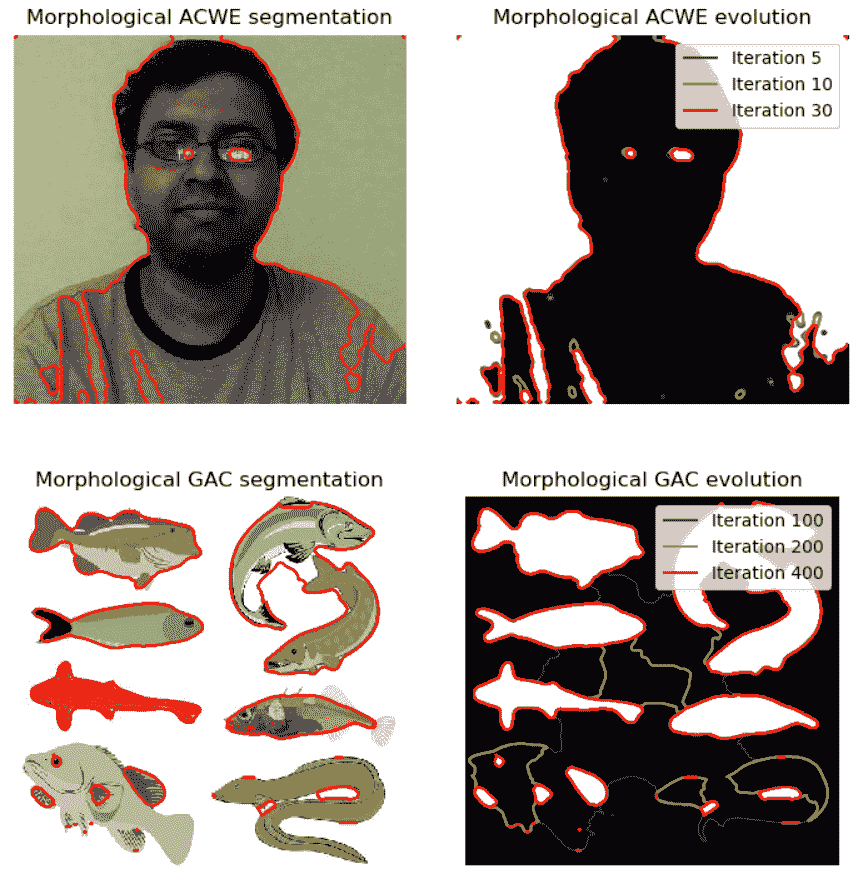# 带 OpenCV 的 grabbut

GrabCut是一种交互式分割方法，使用图论最大流/最小割算法从图像背景中提取前景。在算法开始之前，用户首先需要提供一些提示，在输入图像中大致指定前景区域，尽可能减少交互（例如，在前景区域周围绘制一个矩形）。然后，该算法迭代分割图像以获得最佳结果。在某些情况下，分割可能不是期望的（例如，算法可能已将某些前景区域标记为背景，反之亦然）。

# 问题

1. 使用 Hough 变换从带有scikit-image椭圆的图像中检测椭圆
2. 使用scikit-image转换模块的probabilistic_hough_line()功能从图像中检测线条。它与hough_line()有何不同？
3. 使用scikit-image过滤器模块的try_all_threshold()功能比较不同类型的局部阈值技术，将灰度图像分割为二值图像。
4. 使用ConfidenceConnectedVectorConfidenceConnected算法对使用SimpleITK的 MRI 扫描图像进行分割。
5. 在前景对象周围使用正确的边框，使用 GrabCut 算法分割鲸鱼图像。
6. 使用scikit-image分割模块的random_walker()功能分割图像开始。。。